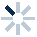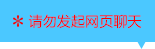| | |

||
APP端下载AndroidiPhone
|
￥56

|
50人点赞
45943人已学习
|

3天无理由退款

3天无理由退款：退款将以超级币形式退至您的超级课堂学习账户，便于您重新选购其他课程。恶意退款将被冻结账号。

• 1、认识了横切、竖切、斜切对直柱体的压强的影响：横切压强变小；竖切压强不变；斜切压强可能变大也可能变小
2、 熟悉了竖切压强不变在题目中的应用，要熟练地在压力，压强，底面积，质量，密度，体积这些物理量之间自由转化，才能快速解题
3、 最后一题的另一种解法很巧妙，通过构造出竖切的情况，就很容易判断出丙和丁的压强大小了
• 1、当叠加体各层进行竖切时，最底层的直柱体对水平面的压强始终不变，为$\rho _{1}gh_{1}$。只需要考虑压强附加值的变化，也就是上方的总重力和最底层的底面积之比的这部分
2、 如果是直柱体叠加，对每一层都进行竖切，则每一项的$\rho gh$部分是不变的，变化的只有底面积比。拿最简单的双层直柱叠加体来说，当两层都进行竖切，则$\rho _{1}gh_{1}$、$\rho _{2}gh_{2}$不变，若$\dfrac{S_{2}}{S_{1}}$变大，则压强变大；$\dfrac{S_{2}}{S_{1}}$变小，则压强变小；$\dfrac{S_{2}}{S_{1}}$不变，则压强不变
• 1、直柱体的竖切并重组主要有两类题型：一类是将物体竖切一部分，再放到剩余部分的上面，从而形成叠加体。此时总重力不变，底面积变小，因而压强变大。这类题的技巧是采用特值法
2、 另一类是切割后互换的题型。底层物体对水平面的压强前后不变，只是上方多了一个物体，所以压强增大的部分就是增加部分的重力与剩余底面积之比
• 1、通过割补法，发现直柱体斜切后，上小下大则压强减小，小于$ρgh$；上大下小则压强增大，大于$ρgh$。记住这个规律，斜切的题目就能马上判断出答案
2、 重点关注了割补法。通过补全或切割出一个中介图形，来实现不规则图形和整体图形的压强比较
3、 最后一道题就巧用割补法中的“补”。把难以比较压强的三角块补成了直柱体，这样只看高度就能判断压强大小了
• 1、在两个压强公式的基础上，比较横切后的压强大小变化时，如果题目存在质量相同的条件，就说明压力相同，适合利用公式$p=\dfrac{F}{S}$来推出更多的大小关系；如果题目有压强的条件，往往更适合利用公式$p=ρgh$
2、 此外，还使用到了极限法这种特殊的技巧
3、 对于横切互换的题目，可以通过比较互换部分质量的大小，来判断压强的变化
• 1、横切的压力和压强的叠加规律：$F_{上}+F_{下}=F$，$p_{上}+p_{下}=p$
2、 利用这两个规律，解决了三道判断横切后压力或压强大小比较的题目，在解题中，同学们始终要注意密度公式和压强公式的灵活运用
• 1、学习同一均匀直柱体横切的压强比例规律
2、 设密度为$ρ$、高度为$h$的直柱体横切掉的高度为$\Delta h$，剩余的高度为$h_{剩}$，则存在比例关系：$\dfrac{\Delta h}{h}=\dfrac{\Delta p}{p}$，$\dfrac{h_{剩}}{h}=\dfrac{p_{剩}}{p}$，$\dfrac{\Delta h}{h_{剩}}=\dfrac{\Delta p}{p_{剩}}$ 。也就是说，对于同一个直柱体，压强的比例就是高度的比例
• 1、对于同一直柱体，高度的比例，就是体积的比例，也就是质量的比例
2、 高度比，体积比，质量比，都等于压强比，即$\dfrac{\Delta h}{h}=\dfrac{\Delta V}{V}=\dfrac{\Delta m}{m}=\dfrac{\Delta p}{p}$，$\dfrac{h_{剩}}{h}=\dfrac{V_{剩}}{V}=\dfrac{m_{剩}}{m}=\dfrac{p_{剩}}{p}$，$\dfrac{\Delta h}{h_{剩}}=\dfrac{\Delta V}{V_{剩}}=\dfrac{\Delta m}{m_{剩}}=\dfrac{\Delta p}{p_{剩}}$
• 1、​横切和竖切规律的综合运用。除了强化了基本的横切竖切规律，还要注意这些题目中运用到的一些技巧，比如逆推反证的技巧，列出比例代数式相互表示和物理代数式化简、代换的技巧，把孔洞看成竖切或横切问题的技巧。这些才是题目的难点，也是同学们要在本节课中学到的新内容

• 115977526896
• 2超级学员950339
• 3影风-品月
• 4超级学员2255184
• 5李京超级棒
• 6超级学员3203240
• 7超级学员3292348
• 8超级学员3297891
• 9超级学员3919003
• 10超级学员4239069204262人在学
￥ 78 ￥ 78202831人在学
￥ 32 ￥ 32204343人在学
￥ 64 ￥ 64203652人在学
￥ 32 ￥ 32

• 0
• 点击分享有好礼
••app端下载关注微信号